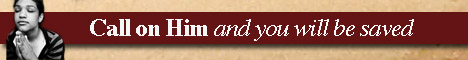Note:  Do not rely on this information. It is very old.

# Triangle

Triangle is literally a figure possessing three angles, but is often defined as a figure bounded by three lines. In plane geometry these lines are straight, and triangles are classified in accordance with the relations between the sides. When all the sides me equal, the triangle is said to be equilateral; if two are equal, the triangle is isosceles; and when all are unequal it is scalene. A triangle possessing one right angle is said to be right-angled, the side opposite the right-angle being known as the hypotenuse. When one angle is greater than a right-angle, the triangle is obtuse-angled; and when all the angles are less than right-angles, it is acute-angled. Since the sum of the three angles is exactly two right-angles, it follows that at least two of the angles must always be acute. Plane is, in reality, based upon the properties of the triangle, since the properties of other figures are generally deduced from those of this simplest figure. The longest side of a triangle is always opposite to the greatest angle, and vice versa; and the connection between the sides and angles forms the basis of the science of trigonometry. The three lines drawn from the vertices to the mid-points of the opposite sides meet in a point, and this point is the centre of gravity of the triangle. The three perpendiculars to the sides drawn from the opposite angles also meet in a point which would be the centre of a circle inscribed in the triangle, while the bisectors of the three angles meet at the centre of the circle which passes through each vertex. If one side of a triangle be produced, the exterior angle which it makes with the adjacent side is equal to the sum of the two interior and opposite angles, and the three exterior angles (formed by producing the three sides in order) are together equal to four right angles. The area of a triangle is equal to half the product of its base, and the perpendicular from the opposite vertex on this base. In a right-angled triangle the square on the hypotenuse is equal to the sums of the squares on the two sides; thus a right-angled triangle can be drawn whose sides are 3,4, and 5 inches respectively, the squares on the sides being 9 and 16 square inches, while that on the hypotenuse is 25 square inches. In spherical trigonometry triangles are considered whose sides are portions of circles, and these are obtained by drawing figures on a sphere. The simplest spherical triangles are those whose sides are porbions of great circles. The geometry of spherical triangles is naturally of great importance, on account of its application in measurements on the earth and in navigation.